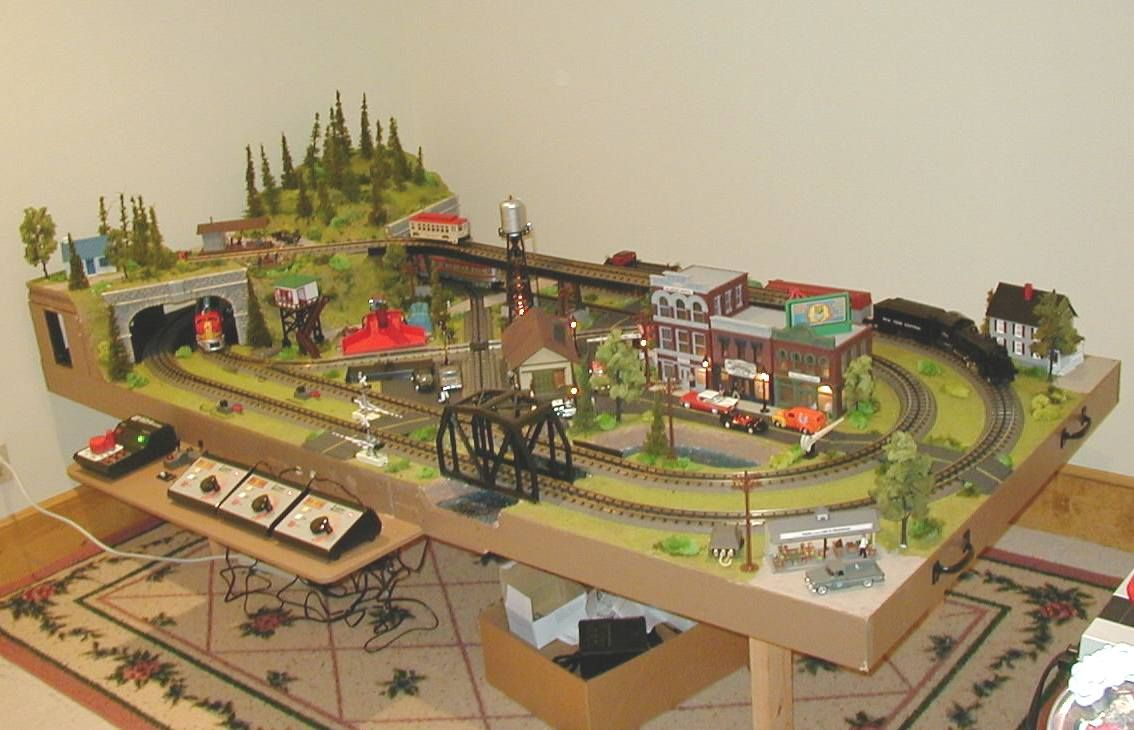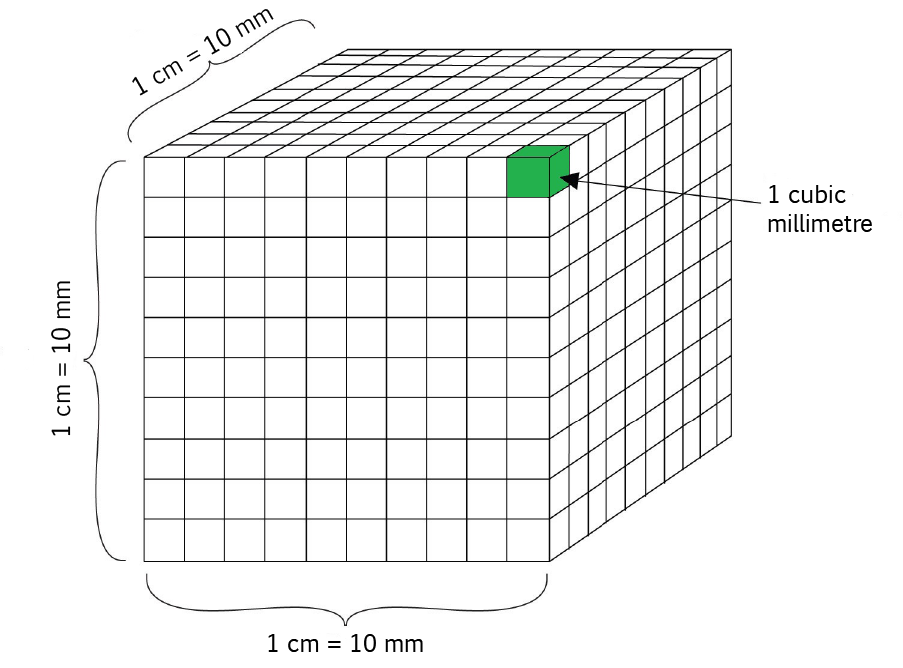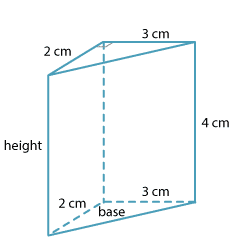# Converting VolumesSam and Jasmin have decided that they'd like to build Christo a layout for his model trains as a birthday present. They've decided to build a mountain, and have calculated that they'll need to carve it out of 124,000 cubic millimetres of styrofoam. However, they've discovered that the styrofoam is sold by the cubic centimetre, so they'll need to convert their volume to a volume in cubic centimetres.

Learning to convert volumes is a very useful skill, and one that we should all spend some time acquiring. In this article we'll look at how we can convert volumes between various cubic units in the metric system. At the end, we'll talk about converting volumes from metric units to Imperial units and vice versa.

## Converting Metric Volumes to other Metric Cubic Units

### Cubic Centimetres to Cubic Millimetres and Back Again

Each centimetre is made up of $10$ millimetres. So, a square with a $1$ centimetre side length has a $10$ millimetre side length. Because the area of a square is equal to its side-length squared, this means that $1$ square centimetre is equal to $10 \times 10 = 100$ square millimetres. But the volume of a cube is equal to its side length cubed, so we need to multiply by $10$ millimetres again to find our conversion for cubic centimetres into cubic millimetres. $1$ cubic centimetre is equal to $10 \times 100 = 1,000$ cubic millimetres as shown in the diagram below.To convert a volume from cubic centimetres to cubic millimetres, you need to multiply it by $1,000$. For example, a volume of $23$ cubic centimetres would convert to $23 \times 1,000 = 23,000$ cubic millimetres.

To convert a volume from cubic millimetres to cubic centimetres, you need to remember that the cubic millimetre is the smaller unit, so you need to divide by $1,000$. So, Jasmin and Sam will need $124,000 \div 1,000 = 124$ cubic centimetres of styrofoam to build Christo's mountain.

### Cubic Metres to Cubic Centimetres and Back Again

Each metre is made up of $100$ centimetres. So, a square with a $1$ metre side length has a $100$ centimetre side length. Because the area of a square is equal to its side-length squared, this means that $1$ square metre is equal to $100 \times 100 = 10,000$ square centimetres. Because the volume of a cube is equal to its side-length cubed, we need to multiply by $100$ again to find that $1$ cubic metre is equal to $10,000 \times 100 = 1,000,000$ cubic centimetres. For example, a rectangular prism with a volume of $27$ cubic metres will have a volume of $27 \times 1,000,000 = 27,000,000$ cubic centimetres.

To convert a volume from cubic centimetres to cubic metres, you need to remember that each cubic metre is made up of $1,000,000$ cubic centimetres, so you need to divide your volume by $1,000,000$. For example, a volume of $4,000,000$ cubic centimetres would become an area of $4,000,000 \div 1,000,000 = 4$ cubic metres.

### Cubic Metres to Cubic Millimetres and Back Again

We know that there are $100$ centimetres in a metre and $10$ millimetres in a centimetre. Combining these two facts together tells us that there are $1,000$ millimetres in a metre. So, a cube with a side length of $1$ metre will have a side length of $1,000$ millimetres. Because the volume of a square is equal to its side-length cubed, this means that $1$ cubic metre is equal to $1,000 \times 1,000 \times 1,000 = 1,000,000,000$ cubic millimetres.

To convert a measurement from cubic metres to cubic millimetres, simply multiply it by $1,000,000,000$. For example, a rectangular prism with a volume of $27$ cubic metres has a volume of of $27 \times 1,000,000,000 = 27,000,000,000$ cubic millimetres.

Sometimes, we need to convert volumes in cubic millimetres to volumes in cubic metres. To do this, remember that cubic millimetres are smaller than cubic metres and divide the number of cubic millimetres by $1,000,000,000$. For example, if a rectangular prism has a volume of $64,000,000,000$ cubic millimetres, it has a volume of $64,000,000,000 \div 1,000,000,000 = 64$ cubic metres.

### Converting Cubic Centimetres to Millilitres and Back Again

This is as particularly easy conversion. One cubic centimetre is equal to one millilitre. So, a volume of $33$ cubic centimetres is equal to a volume of $33$ millilitres, and a volume of $750$ millilitres is equal to a volume of $750$ cubic centimetres.

### Example: Doing Conversions in Geometry ProblemsHere's a problem out of Sam's maths text book that he'd like some help with solving. He's been asked to find the volume of this triangular prism in cubic millimetres.

Well Sam, the first thing you should do is find the volume in cubic centimetres as all the measurements are given in centimetres. Remember that the area of a triangle is equal to half of its base times its height, and the volume of a prism is equal to the area of the base times the height. So, Sam, the volume of your triangular prism is equal to $\dfrac{1}{2} \times 2 \times 3 \times 4 = 12$ cubic centimetres.

Next, multiply this volume by $1,000$ as each cubic centimetre is made up of $1,000$ cubic millimetres. The answer is $12 \times 1,000 = 12,000$ cubic millimetres.

## Converting between Metric and Imperial Volumes.

We can convert between metric and Imperial volumes using similar methods. We just need to know what number to multiply each cubic unit by to give the conversion. Alternatively, if we know the number used to do the length conversion, we can multiply by it three times to find the number used to do the volume conversion.

For example, one foot is $0.3048$ metres. So, one cubic foot is equal to $0.3048 \times 0.3048 \times 0.3048 = 0.0283$ cubic metres. So, to convert a volume of $1,000$ cubic feet to cubic metres, we simply multiply it by $0.0929$ to give $,1000 \text{ cubic feet} = 1,000 \times 0.0283 = 28.3 \text{ cubic metres}$.

As a final example, one inch is the same as $25.4$ millimetres. So, one inch cubed is the same as $25.4 \times 25.4 \times 25.4 = 16,387.064$ cubic millimetres. To convert a volume in cubic inches into a volume in cubic millimetres, simply multiply it by $16,387.064$. For example, a volume of $1,000$ cubic inches is the same as a volume of $1,000 \times 16,387.064 = 16,387,064$ cubic millimetres.

You can do similar conversions between any Imperial unit of volume and any metric unit of volume, and vice versa. You just need to know the conversion factors (numbers to multiply by). Have fun!

### Description

• Metric numbers
• Speed
• Conversions

and several other topics

### Audience

Year 10 or higher, some chapters are suitable for Year 8+ students

### Learning Objectives

Learn about measurement and related topics

Author: Subject Coach
You must be logged in as Student to ask a Question.